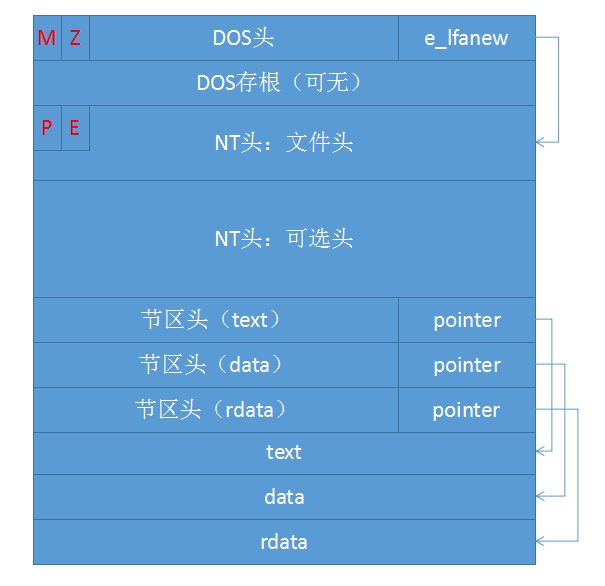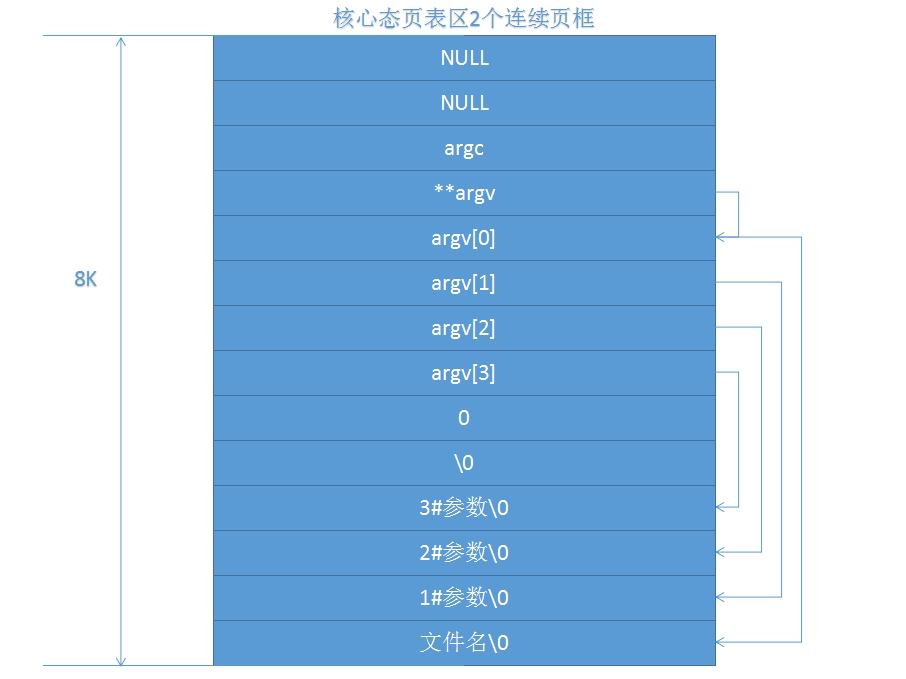• PE 文件格式
• Exec 执行流程

## Portable Executable File (PE)Q

A

Q

A

## Exec

`Exec`仅仅刷新进程的用户态图像，即代码段，数据段，堆栈段。进程核心态图像不变，依旧保持`fork`之后的值。

``````// interrupt/SystemCall.cpp
/*	11 = exec	count = 2	*/
int SystemCall::Sys_Exec()
{
ProcessManager& procMgr = Kernel::Instance().GetProcessManager();
procMgr.Exec();

return 0;	/* GCC likes it ! */
}
``````

• 找到要打开的文件
• 检查如果超过大小限制就不打开
• 检查有没有权限打开
• 解析 PE 格式，根据头部信息读入数据段
• 查看 text 块是不是已经在内存，不是则读入代码段
• 分配堆和栈
• 建立相对地址映射表
• 把之前的参数放入用户态的栈上
• EIP 设置为它的入口地址，执行程序

### Stage 1 寻找文件，权限检测

``````pInode = fileMgr.NameI(FileManager::NextChar, FileManager::OPEN);
``````

``````while( this->ExeCnt >= NEXEC )
{
u.u_procp->Sleep((unsigned long)&ExeCnt, ProcessManager::EXPRI);
}
this->ExeCnt++;
``````

``````if ( fileMgr.Access(pInode, Inode::IEXEC) || (pInode->i_mode & Inode::IFMT) != 0 )
{
fileMgr.m_InodeTable->IPut(pInode);
if ( this->ExeCnt >= NEXEC )
{
WakeUpAll((unsigned long)&ExeCnt);
}
this->ExeCnt--;
return;
}
``````

### Stage 2 解析文件头

``````PEParser parser;
{
fileMgr.m_InodeTable->IPut(pInode);
return;
}
// 正文段地址及长度
u.u_MemoryDescriptor.m_TextSize = parser.TextSize;
// 数据段地址及长度
u.u_MemoryDescriptor.m_DataSize = parser.DataSize;
// 堆栈段初始化长度
u.u_MemoryDescriptor.m_StackSize = parser.StackSize;
``````

``````if ( parser.TextSize + parser.DataSize + parser.StackSize  + PageManager::PAGE_SIZE > MemoryDescriptor::USER_SPACE_SIZE - parser.TextAddress)
{
fileMgr.m_InodeTable->IPut(pInode);
u.u_error = User::ENOMEM;
return;
}
``````

### Stage 3 保护用户栈中 exec 参数

``````int allocLength = (parser.StackSize + PageManager::PAGE_SIZE * 2 - 1) >> 13 << 13;
unsigned long fakeStack = kernelPgMgr.AllocMemory(allocLength);
``````

``````int argc = u.u_arg;
char** argv = (char **)u.u_arg;
unsigned int esp = MemoryDescriptor::USER_SPACE_SIZE;
unsigned long desAddress = fakeStack + allocLength + 0xC0000000;
int length;
for (int i = 0; i < argc; i++ )
{
length = 0;
while( NULL != argv[i][length] )
{
length++;
}
Utility::MemCopy((unsigned long)argv[i], desAddress, length + 1);
esp = esp - (length + 1);
argv[i] = (char *)esp;
}
``````

``````desAddress = desAddress & 0xFFFFFFF0;
esp = esp & 0xFFFFFFF0;
int endValue = 0;
esp -= sizeof(endValue);

esp -= argc * sizeof(int);
Utility::MemCopy((unsigned long)argv, desAddress, argc * sizeof(int));
``````

``````endValue = esp;
esp -= sizeof(int);

esp -= sizeof(int);
``````### Stage 4 缩小空间到 PPDA 和 proc

``````if ( u.u_procp->p_textp != NULL )
{
u.u_procp->p_textp->XFree();
u.u_procp->p_textp = NULL;
}
``````

``````// USIZE 正是 PPDA 区的大小
u.u_procp->Expand(ProcessManager::USIZE);
``````

### Stage 5 分配代码段空间并写 text 块

``````pText = NULL;
for ( int i = 0; i < ProcessManager::NTEXT; i++ )
{
if ( NULL == this->text[i].x_iptr )
{
if ( NULL == pText )
{
pText = &(this->text[i]);
}
}
else if ( pInode == this->text[i].x_iptr )
{
this->text[i].x_count++;
this->text[i].x_ccount++;
u.u_procp->p_textp = &(this->text[i]);
pText = NULL;
break;
}
}
``````

``````if ( NULL != pText )
{
pInode->i_count++;
pText->x_ccount = 1;
pText->x_count = 1;
pText->x_iptr = pInode;
pText->x_size = u.u_MemoryDescriptor.m_TextSize;
u.u_procp->p_textp = pText;
}
else
{
pText = u.u_procp->p_textp;
sharedText = 1;
}
``````

### Stage 6 建立地址映射

``````unsigned int newSize = ProcessManager::USIZE + u.u_MemoryDescriptor.m_DataSize + u.u_MemoryDescriptor.m_StackSize;
u.u_procp->Expand(newSize);
``````

``````u.u_MemoryDescriptor.EstablishUserPageTable(parser.TextAddress, parser.TextSize, parser.DataAddress, parser.DataSize, parser.StackSize);
``````

### Stage 7 创建新用户态图像

``````parser.Relocate(pInode, sharedText);
``````

``````if(sharedText == 0)
{
u.u_procp->p_flag |= Process::SLOCK;
u.u_procp->p_flag &= ~Process::SLOCK;
}
``````

### Stage 8 准备命令行参数

``````Utility::MemCopy(fakeStack + allocLength - parser.StackSize | 0xC0000000, MemoryDescriptor::USER_SPACE_SIZE - parser.StackSize, parser.StackSize);
kernelPgMgr.FreeMemory(allocLength, fakeStack);
``````

### Stage 9 拷贝 runtime() 和 SignalHandler()

`runtime()``SignalHandler()`拷到进程线性地址的起始处`0x00000000h`

``````unsigned char* runtimeSrc = (unsigned char*)runtime;
unsigned char* runtimeDst = 0x00000000;
for (unsigned int i = 0; i < (unsigned long)ExecShell - (unsigned long)runtime; i++)
{
*runtimeDst++ = *runtimeSrc++;
}
``````

``````extern "C" void runtime()
{
__asm__ __volatile__("	leave;	\
movl %%esp, %%ebp;	\
call *%%eax;		\
movl \$1, %%eax;	\
movl \$0, %%ebx;	\
int \$0x80"::);
}
``````

### Stage 10 扫尾，准备进入用户态

``````u.u_ar0[User::EAX] = parser.EntryPointAddress;
``````

### Stage 11 进入用户态，调用 main 函数

``````struct pt_context* pContext = (struct pt_context *)u.u_arg;
pContext->eip = 0x00000000;
pContext->xcs = Machine::USER_CODE_SEGMENT_SELECTOR;
pContext->eflags = 0x200;
pContext->esp = esp;
pContext->xss = Machine::USER_DATA_SEGMENT_SELECTOR;
``````

`runtime`是一个 C 语言函数，所以前两条指令是编译器添加的栈帧构造指令：

``````pushl %ebp
movl %esp, %ebp
``````

``````leave
``````

``````call *%%eax
``````